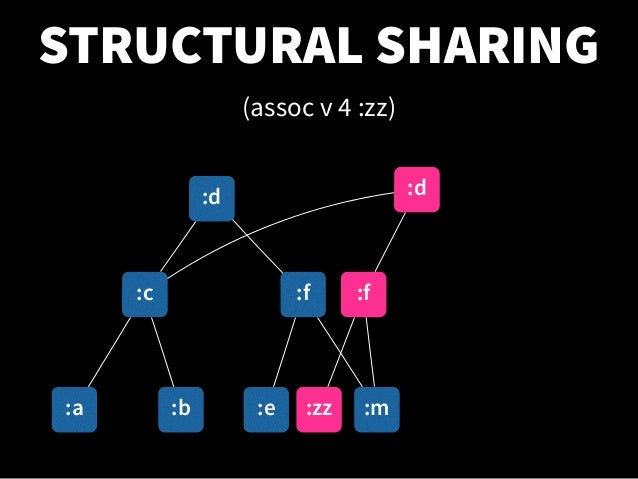# 函数式编程入不了门

2020-05-23 08:00:00# What is it and why use it？

``````// 以下代码描述了翻转二叉树的过程
function invertTree(node) {
if (node == null) return null

node.left = invertTree(node.right)
node.right = invertTree(node.left)

return node
}
``````
``````// 以下代码体现了如何从旧树得到一颗新树
const invertTree = (node) => {
return node == null
? null
: new Tree(node.value, invertTree(node.right), invertTree(node.left))
}
``````

1声明式编程，不拘泥于细节，代码更清晰较难学习与上手
2纯函数职责更小，利于测试与维护需要更高层的抽象思维
3鼓励复杂任务的分解，代码可重用程度变高

## Purity

``````const greet = (name) => `Hi, \${name}`

greet('Brianne') // 'Hi, Brianne'``````
``````window.name = 'Brianne'

const greet = () => `Hi, \${window.name}`

greet() // "Hi, Brianne"``````
``````let greeting

const greet = (name) => {
greeting = `Hi, \${name}`
}

greet('Brianne')
greeting // "Hi, Brianne"``````
``````Date.now() // ?
Math.random() // ?``````

### Referential Transparency

``````multiply(sum(1, 2),sum(1, 3)) // -> 12

// 函数的调用可以直接被返回值替换

multiply(3, 4)) // -> 12``````

## HOF❗️❗️❗️

JS 中函数是一等公民，可以像其他的变量一样被传递。

``````map / filter / reduce

map(v -> v)
filter(v -> !v)

.map(1) = 
.map(() => 2) ``````

## Immutable data

``````// number / string / boolean 都是不可变数据
let str = 'functional programming'
// 返回新的状态，而不是对旧状态的修改
str = 'declarative programming'

let arr = 

arr = ''``````
1. Object.freeze - 只是浅冻结, 更深层的冻结需要递归查找耗损性能2. CoW - 不断复制造成性能损耗

Copy-on-write

Lenses

``````interface Lens<S, A> {
get(s: S): A
set(a: A, s: S): S
}

}

// => {num: 23, name: "high street"}
address.set({ num: 23, name: 'main street' }, a1)
// => {city: "london", street: {num: 23, name: "main street"}}``````

Introduction to optics: lenses and prisms

3. Structure SharingPersistent data structure

JavaScript 中支持 Structure Sharing 的工具：

immerjs/immer

Immutable.js

# Currying

currying 的概念很简单：对于多形参的函数，调用时只传递给它一部分参数，让它返回一个函数去处理剩下的参数。

``````const add = function (x) {
return function (y) {
return x + y
}
}

add = (x, y) => x + y

increment(2)
// 3

// 12``````

# Combinators

compose（Redux / Chain of Responsibility）

``compose(fc, fb, fa)('arg') = fc(fb(fa('arg')))``

pipe

``pipe(fa, fb, fc) = fc(fb(fa('arg')))``

tap

``````// tap :: (x -> *) -> x -> x
tap((x) => {
/* do anything */
})(x) === x
``````

seq

``seq(fa, fb, fc)(x) === x``

alt

``````alt(returnFalse, returnOther)(x)

{
const returnValue = returnFalse(x)

return returnValue || returnOther(x)
}
``````

forkJoin

``````fork(last, f1, f2)(x)

{
const r1 = f1(x),
r2 = f2(x)

return last(r1, r2)
}
``````

## Side-effect

https://inews.gtimg.com/newsapp_bt/0/10524648136/1000

https://img3.doubanio.com/view/photo/l/public/p2589766490.webp

null 是一个价值数十亿美金的错误Error / Exception

1. 难以与其他函数组合与链接
2. 会引起副作用
3. 不能只关注函数的返回值，还需要负责异常的处理
4. 多个异常时会出现嵌套的 try ... catch..

https://img1.doubanio.com/view/photo/l/public/p2589766489.webp

### 容器### Functor → 对操作容器内数据行为的抽象1. Functor 必须是无副作用的

``new Functor('x').map((v) => v) // -> Functor('x')``
2. 必须可以与其他函数组合

``new Functor(2).map(pipe(plus3, tap(log))) // -> Functor('5')``

JS 中 Array 就是一个 Functor。1. of 函数，可以将值存入容器中
2. map 函数，与容器内的值交互
3. join（flatten），将嵌套的容器拍扁
4. chain，组合了 map 与 join# 优化

## 使用函数组合子避免不必要的计算

``````const showStudent = pipe(
alt(findStudent, createNewStudent),
append('#student-info')
)
``````

## 🤷‍♂️🤷‍♂️🤷‍♂️ shortcut fusion 针对于函数式链的优化

``````const _ = require('lodash')
const { compose, tap } = require('ramda')

const square = compose(
(x) => Math.pow(x, 2),
tap((a) => console.log('square', a))
)

const isEven = compose(
tap(() => console.log('even')),
(x) => x % 2 === 0
)

const numbers = _.range(200)
console.log(numbers)
const result = _.chain(numbers).map(square).filter(isEven).take(3).value()

console.log(result)``````

## 避免过多层级的 currying# 难点

1. FP 算是一门历史悠久的学科，它始于数学家的 lambda 演算，一开始就与数学（范畴论）密不可分，一开始学习会有特别多的概念不清晰。
2. 就算有了些理论基础，一开始实际动手写 FP 代码时，还没有形成函数式思维，也比较困难，可能这也是为什么 lisp 与 haskell 等语言火不起来的原因吧。
3. 千万不要被教条束缚，FP 与 OO 并不是非此即彼的关系，他们可以相辅相成。最重要的是我们要知道我们写的是什么？我们代码在哪里可能会出现问题？

``````> OO 通过封装变化使得代码更容易理解
FP 通过最小化变化使得代码更容易理解

**你可以把副作用关起来，但不要给自己的思想设限。**``````

https://img1.doubanio.com/view/photo/l/public/p2583893058.webp